# sklearn.datasets.load_iris¶

Load and return the iris dataset (classification).

The iris dataset is a classic and very easy multi-class classification dataset.

 Classes 3 Samples per class 50 Samples total 150 Dimensionality 4 Features real, positive

Read more in the User Guide.

Parameters:
return_X_ybool, default=False

If True, returns (data, target) instead of a Bunch object. See below for more information about the data and target object.

New in version 0.18.

as_framebool, default=False

If True, the data is a pandas DataFrame including columns with appropriate dtypes (numeric). The target is a pandas DataFrame or Series depending on the number of target columns. If return_X_y is True, then (data, target) will be pandas DataFrames or Series as described below.

New in version 0.23.

Returns:
dataBunch

Dictionary-like object, with the following attributes.

data{ndarray, dataframe} of shape (150, 4)

The data matrix. If as_frame=True, data will be a pandas DataFrame.

target: {ndarray, Series} of shape (150,)

The classification target. If as_frame=True, target will be a pandas Series.

feature_names: list

The names of the dataset columns.

target_names: list

The names of target classes.

frame: DataFrame of shape (150, 5)

Only present when as_frame=True. DataFrame with data and target.

New in version 0.23.

DESCR: str

The full description of the dataset.

filename: str

The path to the location of the data.

New in version 0.20.

(data, target)tuple if return_X_y is True

A tuple of two ndarray. The first containing a 2D array of shape (n_samples, n_features) with each row representing one sample and each column representing the features. The second ndarray of shape (n_samples,) containing the target samples.

New in version 0.18.

Notes

Changed in version 0.20: Fixed two wrong data points according to Fisher’s paper. The new version is the same as in R, but not as in the UCI Machine Learning Repository.

Examples

Let’s say you are interested in the samples 10, 25, and 50, and want to know their class name.

>>> from sklearn.datasets import load_iris
>>> data = load_iris()
>>> data.target[[10, 25, 50]]
array([0, 0, 1])
>>> list(data.target_names)
['setosa', 'versicolor', 'virginica']


## Examples using sklearn.datasets.load_iris¶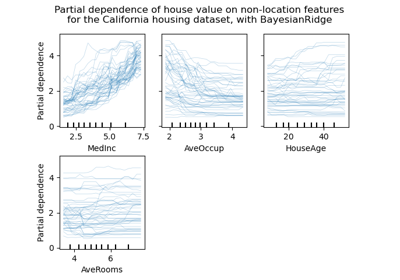Release Highlights for scikit-learn 0.24

Release Highlights for scikit-learn 0.24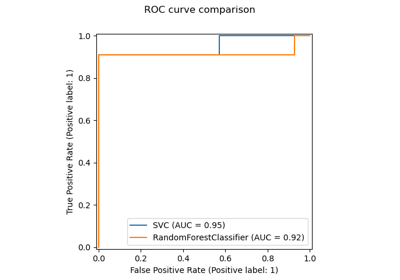Release Highlights for scikit-learn 0.22

Release Highlights for scikit-learn 0.22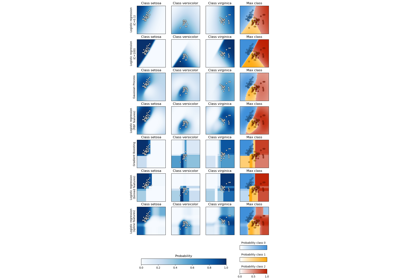Plot classification probability

Plot classification probabilityK-means Clustering

K-means Clustering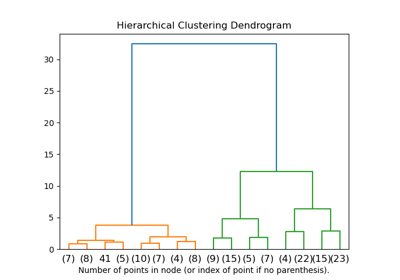Plot Hierarchical Clustering Dendrogram

Plot Hierarchical Clustering DendrogramThe Iris Dataset

The Iris Dataset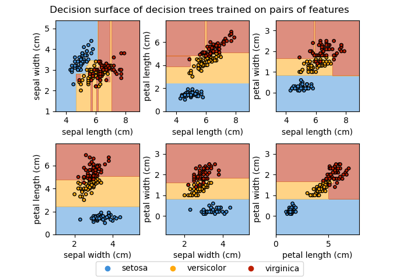Plot the decision surface of decision trees trained on the iris dataset

Plot the decision surface of decision trees trained on the iris dataset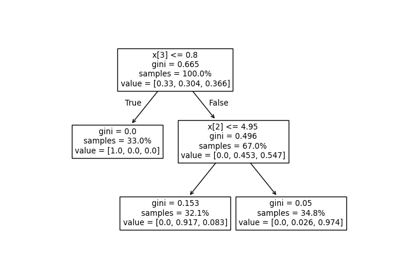Understanding the decision tree structure

Understanding the decision tree structure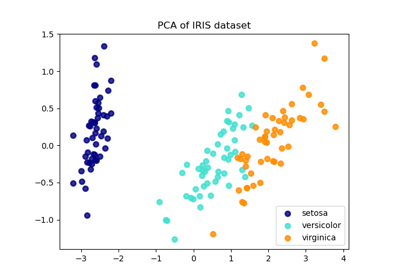Comparison of LDA and PCA 2D projection of Iris dataset

Comparison of LDA and PCA 2D projection of Iris dataset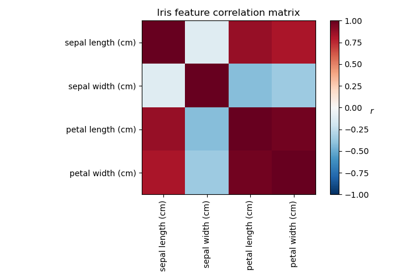Factor Analysis (with rotation) to visualize patterns

Factor Analysis (with rotation) to visualize patterns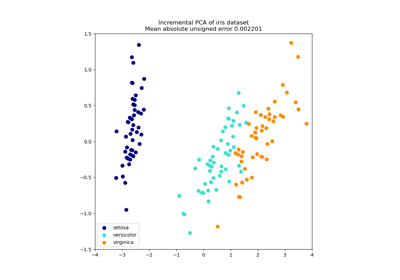Incremental PCA

Incremental PCA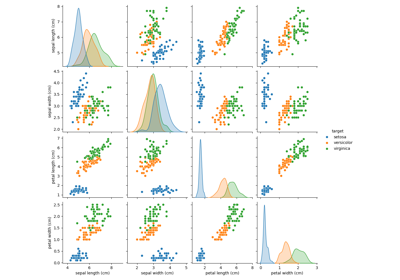PCA example with Iris Data-set

PCA example with Iris Data-set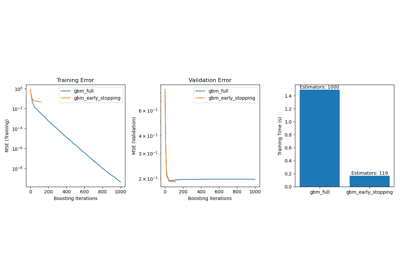Early stopping of Gradient Boosting

Early stopping of Gradient Boosting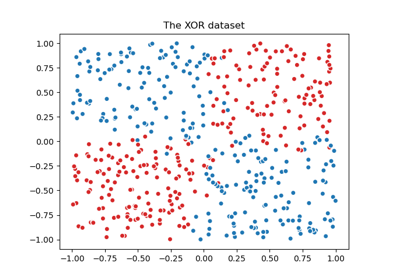Plot the decision boundaries of a VotingClassifier

Plot the decision boundaries of a VotingClassifier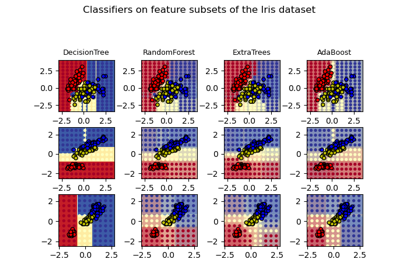Plot the decision surfaces of ensembles of trees on the iris dataset

Plot the decision surfaces of ensembles of trees on the iris dataset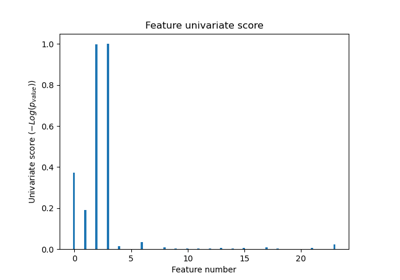Univariate Feature Selection

Univariate Feature Selection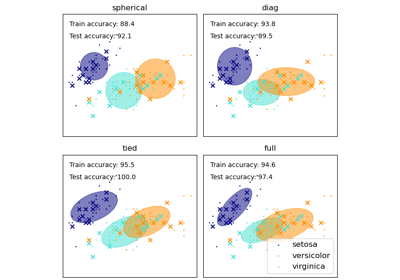GMM covariances

GMM covariances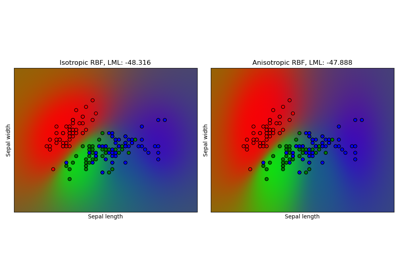Gaussian process classification (GPC) on iris dataset

Gaussian process classification (GPC) on iris datasetLogistic Regression 3-class Classifier

Logistic Regression 3-class Classifier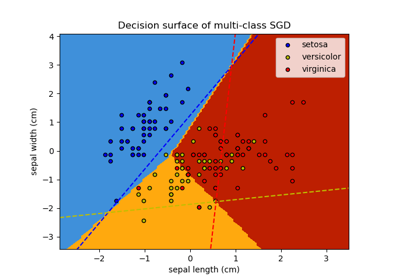Plot multi-class SGD on the iris dataset

Plot multi-class SGD on the iris dataset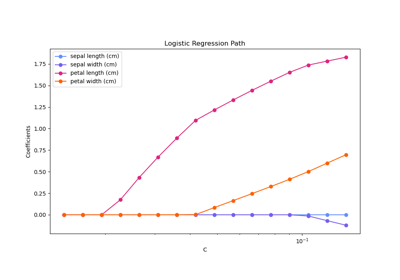Regularization path of L1- Logistic Regression

Regularization path of L1- Logistic Regression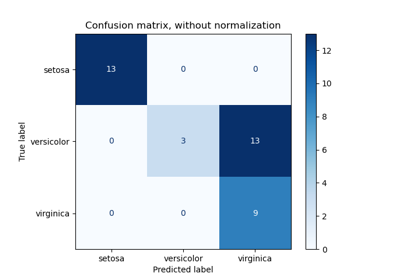Confusion matrix

Confusion matrix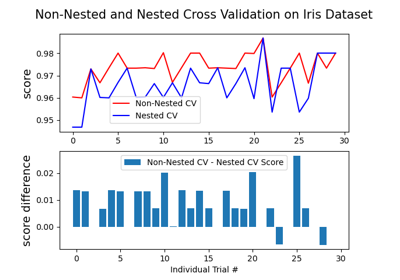Nested versus non-nested cross-validation

Nested versus non-nested cross-validation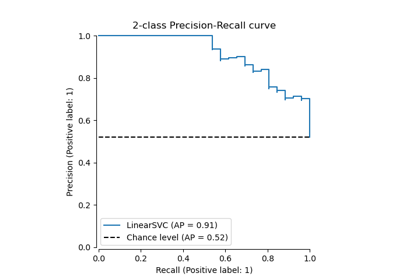Precision-Recall

Precision-Recall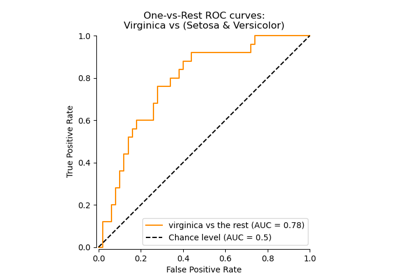Receiver Operating Characteristic (ROC)

Receiver Operating Characteristic (ROC)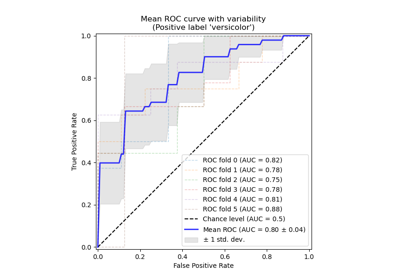Receiver Operating Characteristic (ROC) with cross validation

Receiver Operating Characteristic (ROC) with cross validation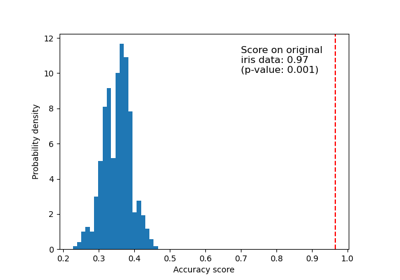Test with permutations the significance of a classification score

Test with permutations the significance of a classification score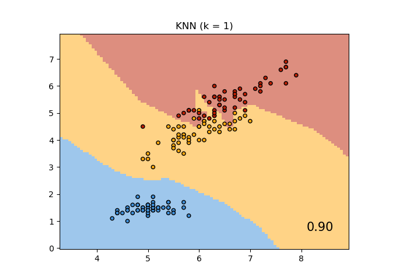Comparing Nearest Neighbors with and without Neighborhood Components Analysis

Comparing Nearest Neighbors with and without Neighborhood Components Analysis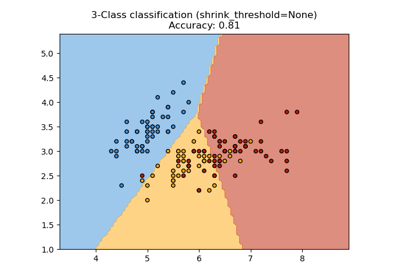Nearest Centroid Classification

Nearest Centroid Classification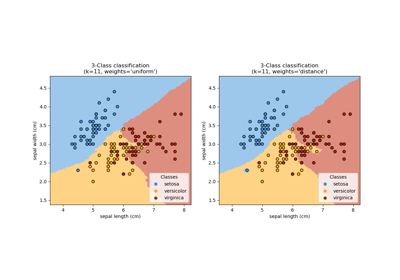Nearest Neighbors Classification

Nearest Neighbors Classification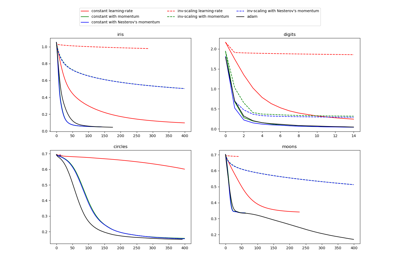Compare Stochastic learning strategies for MLPClassifier

Compare Stochastic learning strategies for MLPClassifierConcatenating multiple feature extraction methods

Concatenating multiple feature extraction methods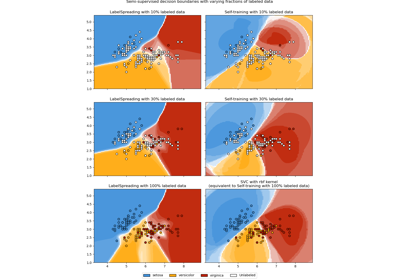Decision boundary of semi-supervised classifiers versus SVM on the Iris dataset

Decision boundary of semi-supervised classifiers versus SVM on the Iris dataset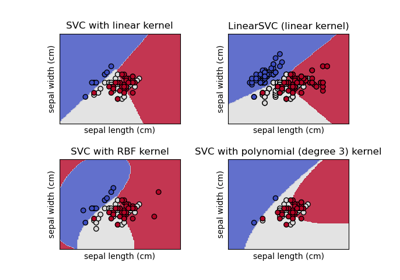Plot different SVM classifiers in the iris dataset

Plot different SVM classifiers in the iris dataset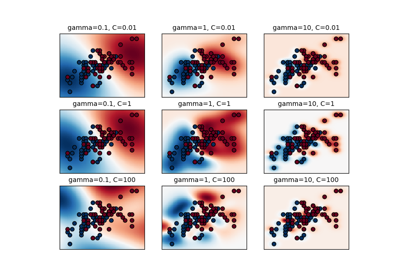RBF SVM parameters

RBF SVM parameters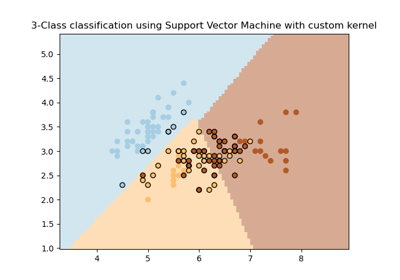SVM with custom kernel

SVM with custom kernel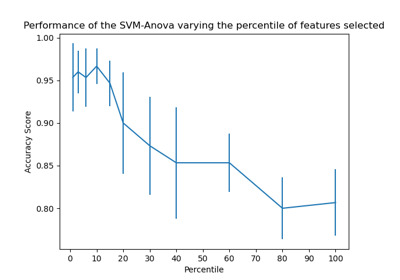SVM-Anova: SVM with univariate feature selection

SVM-Anova: SVM with univariate feature selectionSVM Exercise

SVM Exercise